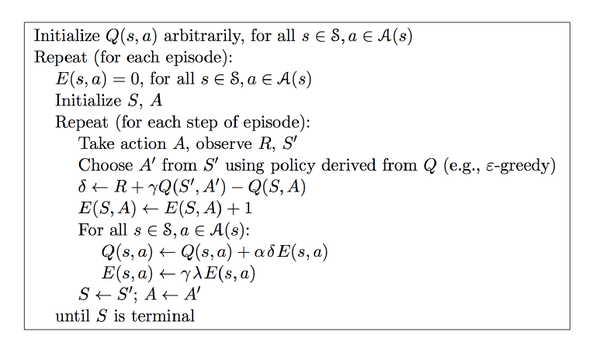## 0x01 基础概念

• 学习&规划

前者是指智能体未知环境模型，需要不断与环境进行交互，不断改进策略

后者是指已知大部分环境模型，智能体不再与环境进行交互，根据状态转移概率和回报，不断进改进策略

• 探索&利用：

探索未曾尝试的决策，主要体现在强化学习算法中的随机策略。

利用已收集的知识，主要体现在一些算法的贪心策略。

• 预测和控制

又叫评估与改善。

分别对应强化学习的打分阶段和策略改善阶段。

## 0x02 马尔可夫决策

$V_{\pi}(s) = \mathbb{E}_{\pi}[G_t|S_t=s] = \mathbb{E}_{\pi}[R_{t+1}+\gamma R_{t+2}+...|S_t=s]$

\begin{aligned} V_{\pi}(s) =& \mathbb{E}_{\pi}[G_t|S_t=s] \\ =& \mathbb{E}_{\pi}[R_{t+1}+\gamma R_{t+2}+\gamma ^2R_{t+3}+...|S_t=s]\\ =& \mathbb{E}_{\pi}[R_{t+1}+\gamma (R_{t+2}+\gamma R_{t+3}...)|S_t=s]\\ =& \mathbb{E}_{\pi}[R_{t+1}+\gamma G_{t+1}|S_t=s]\\ =& \mathbb{E}_{\pi}[R_{t+1}+\gamma V_{\pi}(S_{t+1})|S_t=s]\\ \end{aligned}

\begin{aligned} Q_{\pi}(s,a) =& \mathbb{E}_{\pi}[G_t|S_t=s,A_t=a] \\ =& \mathbb{E}_{\pi}[R_{t+1}+\gamma Q_{\pi}(S_{t+1},A_{t+1})|S_t=s,A_t=a]\\ \end{aligned}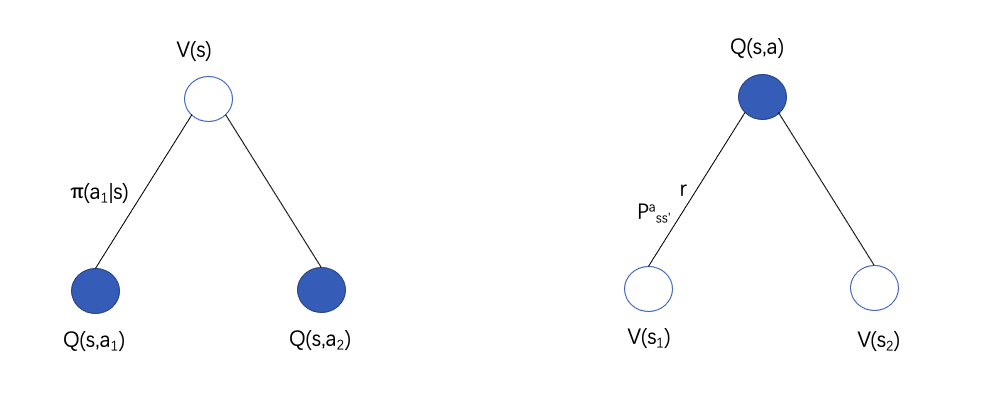$V_{\pi}(s)=\sum_{a\in A}\pi(a|s)Q_{\pi}(s,a)$

$Q_{\pi}(s,a)=R_{s}^a + \gamma \sum_{s' \in S}P^a_{ss'}V_{\pi}(s')$

$V_{\pi}(s) = \sum_{a\in A} \pi(a|s)(R_{s}^a + \gamma \sum_{s' \in S}P^a_{ss'}V_{\pi}(s'))$

$Q_{\pi}(s,a) =R_{s}^a + \gamma \sum_{s' \in S}P^a_{ss'}\sum_{a'\in A}\pi(a'|s')Q_{\pi}(s',a')$

## 0x04 蒙特卡罗 MC

$$

$$

• 初次访问：相同状态的累计回报只计算第一次
• 每次访问：每次经过都计算

$V(s)=\frac{G_{12}+G_{2k}+...}{N(s)}$

MC的主要形式：估计行为值函数Q代替估计值函数V

$Q(s_1,a_1)=\frac{G_{12}+...}{N(s_1,a_1)}$

$Q(s_t,a_t) \leftarrow Q(s_t,a_t) + \alpha (G_t-Q(s_t,a_t))$

## 0x05 时序差分 TD

MC计算的时候需要累积回报均值$G_t$，这就需要知道完整的轨迹（到终止状态）

$V_{\pi}(S_t) = \mathbb{E}_{\pi}[G_t|S_t=s] = \mathbb{E}_{\pi}[R_{t+1}+\gamma V(S_{t+1})|S_t=s]$

$V(s_t)\leftarrow V(s_t) + \alpha(R_{t+1}+\gamma V(s_{t+1})-V(s_t))$

### Saras算法：

$Q(s,a) \leftarrow Q(s,a)+\alpha(R+\gamma Q(s',a')-Q(s,a))$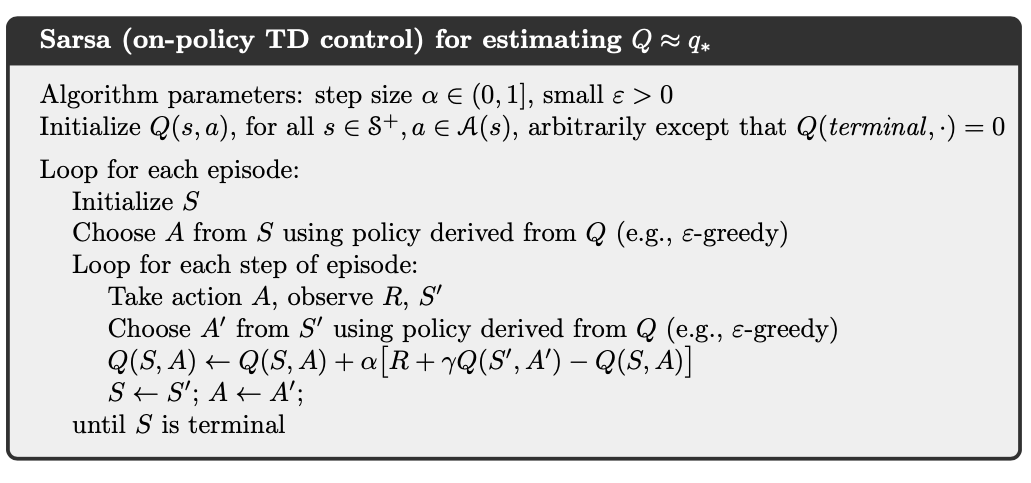### Q-Learning算法：

$Q(s,a) \leftarrow Q(s,a)+\alpha(R+\gamma\ \mathop{max}_{a'} Q(s',a')-Q(s,a))$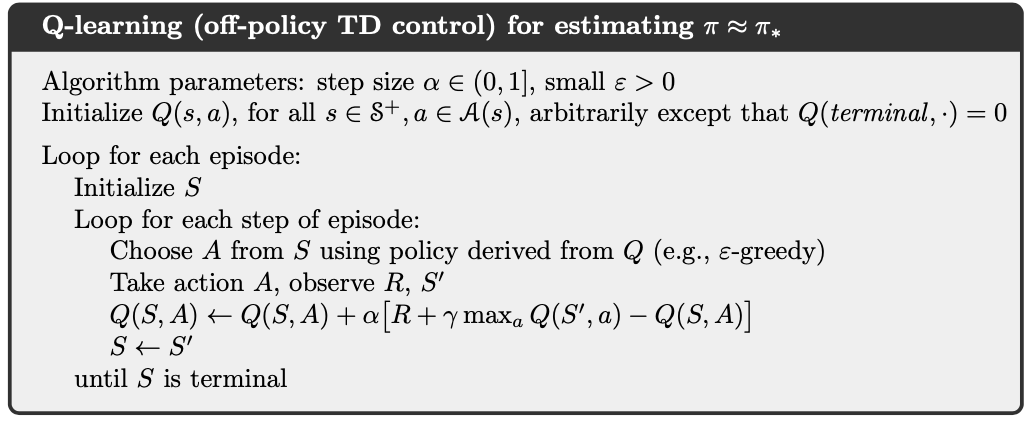## 0x06 资格迹

MC和TD的一个区别是，基于当前状态往未来看的距离不同。

MC：一直观测到结束，整个长度记为$N$

TD: 长度是1，只参考了下一个状态的状态值。

$G_t^n = r_{t+1}+\gamma r_{t+2} +\gamma^2 r_{t+3}+...+\gamma^{n-1}r_{t+n}+\gamma^nV(s_{t+n})$

• 前向视角：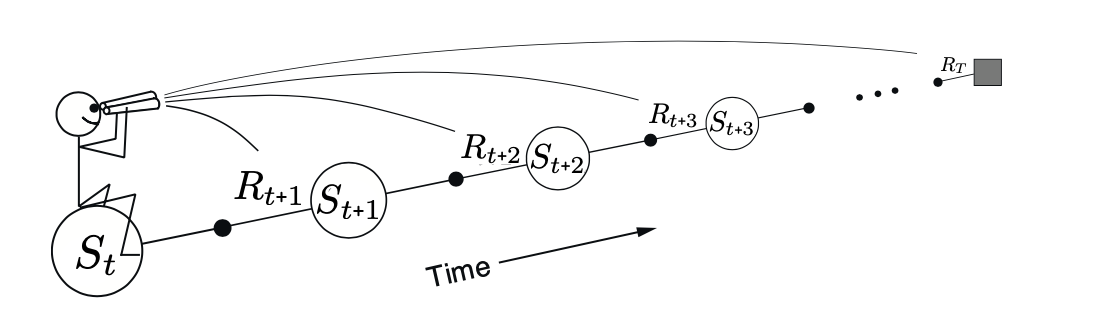​ 前向视角又叫理论视角，使用n个值来更新状态值，保证其权值和为1(资格迹的体现)。

• 后向视角：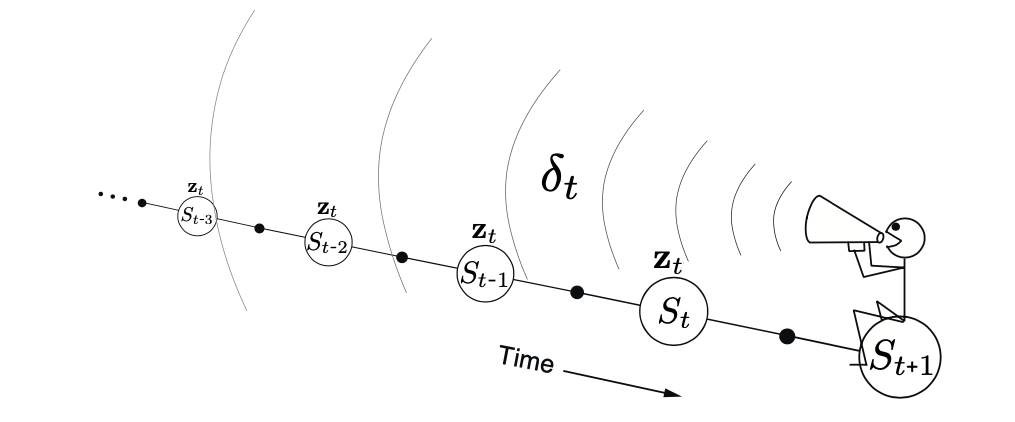在后向视角中，资格迹就是进行资格进行分配，也可以理解成权值的一种分配情况。

### TD($\lambda$)前向算法

$G_{t}^{\lambda} = (1-\lambda)\sum_{n=1}^{\infin} \lambda^{n-1}G^n_t$

$G_{t}^{\lambda} = (1-\lambda)\sum_{n=1}^{T-t-1} \lambda^{n-1}G^n_t+\lambda^{T-t-1}G_t$

$V(s_t) \leftarrow V(s_t) + \alpha(G^{\lambda}_t - V(s_t))$

### TD($\lambda$)后向算法

$t$时刻的状态$s$对应的资格迹，记为$E_t(s)$:

$E_0(s)=0$

$E_t(s) = \gamma \lambda E_{t-1}(s) + I(S_t=s)$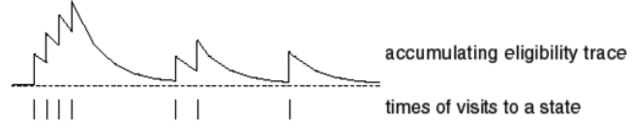\begin{aligned} E_t(S) = \left\{ \begin{array}{l} \gamma \lambda E_{t-1}(s),s_t\neq s\\ 1,s_t=s \end{array} \right. \end{aligned}

TD误差更新如下:

$\delta_t = R_{t+1} + \gamma V_t(S_{t+1})-V_t(S_t)$

$V(s) \leftarrow V(s) + \alpha\delta_tE_t(s)$

### Sarsa($\lambda$) (后向)算法：